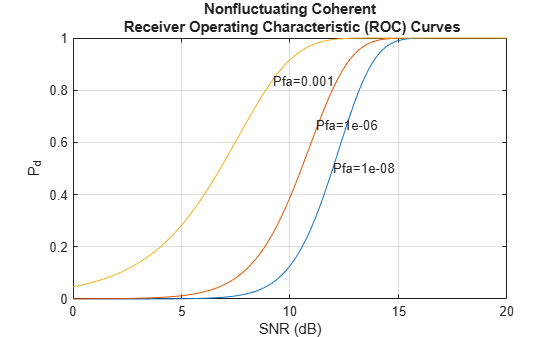Main Content

# rocpfa

Receiver operating characteristic curves by false-alarm probability

## Syntax

```[Pd,SNR] = rocpfa(Pfa) [Pd,SNR] = rocpfa(Pfa,Name,Value) rocpfa(...) ```

## Description

```[Pd,SNR] = rocpfa(Pfa)``` returns the single-pulse detection probabilities, `Pd`, and required SNR values, `SNR`, for the false-alarm probabilities in the row or column vector `Pfa`. By default, for each false-alarm probability, the detection probabilities are computed for 101 equally spaced SNR values between 0 and 20 dB. The ROC curve is constructed assuming a single pulse in coherent receiver with a nonfluctuating target.

```[Pd,SNR] = rocpfa(Pfa,Name,Value)``` returns detection probabilities and SNR values with additional options specified by one or more `Name,Value` pair arguments.

`rocpfa(...)` plots the ROC curves.

## Input Arguments

 `Pfa` False-alarm probabilities in a row or column vector.

### Name-Value Pair Arguments

Specify optional comma-separated pairs of `Name,Value` arguments. `Name` is the argument name and `Value` is the corresponding value. `Name` must appear inside quotes. You can specify several name and value pair arguments in any order as `Name1,Value1,...,NameN,ValueN`.

 `'MaxSNR'` Maximum SNR to include in the ROC calculation. Default: `20` `'MinSNR'` Minimum SNR to include in the ROC calculation. Default: `0` `'NumPoints'` Number of SNR values to use when calculating the ROC curves. The actual values are equally spaced between `MinSNR` and `MaxSNR`. Default: `101` `'NumPulses'` Number of pulses to integrate when calculating the ROC curves. A value of `1` indicates no pulse integration. Default: `1` `'SignalType'` This property specifies the type of received signal or, equivalently, the probability density functions (PDF) used to compute the ROC. Valid values are: `'Real'`, `'NonfluctuatingCoherent'`, `'NonfluctuatingNoncoherent'`, `'Swerling1'`, `'Swerling2'`, `'Swerling3'`, and `'Swerling4'`. Values are not case sensitive. The `'NonfluctuatingCoherent'` signal type assumes that the noise in the received signal is a complex-valued, Gaussian random variable. This variable has independent zero-mean real and imaginary parts each with variance σ2/2 under the null hypothesis. In the case of a single pulse in a coherent receiver with complex white Gaussian noise, the probability of detection, PD, for a given false-alarm probability, PFA is: `${P}_{D}=\frac{1}{2}\text{erfc}\left({\text{erfc}}^{-1}\left(2{P}_{FA}\right)-\sqrt{\chi }\right)$`where `erfc` and `erfc-1` are the complementary error function and that function’s inverse, and χ is the SNR not expressed in decibels. For details about the other supported signal types, see . Default: `'NonfluctuatingCoherent'`

## Output Arguments

 `Pd` Detection probabilities corresponding to the false-alarm probabilities. For each false-alarm probability in `Pfa`, `Pd` contains one column of detection probabilities. `SNR` Signal-to-noise ratios in a column vector. By default, the SNR values are 101 equally spaced values between 0 and 20. To change the range of SNR values, use the optional `MinSNR` or `MaxSNR` input argument. To change the number of SNR values, use the optional `NumPoints` input argument.

## Examples

collapse all

Plot ROC curves for false-alarm probabilities of 1e-8, 1e-6, and 1e-3, assuming no pulse integration.

```Pfa = [1e-8 1e-6 1e-3]; rocpfa(Pfa,'SignalType','NonfluctuatingCoherent')```## References

 Richards, M. A. Fundamentals of Radar Signal Processing. New York: McGraw-Hill, 2005, pp 298–336.

## See Also

Introduced in R2011a

## Support

#### Exploring Hybrid Beamforming Architectures for 5G Systems

Download white paper